# Transform EEG data using current source density (CSD)¶

This script shows an example of how to use CSD :footcitePerrinEtAl1987,PerrinEtAl1989,Cohen2014,KayserTenke2015. CSD takes the spatial Laplacian of the sensor signal (derivative in both x and y). It does what a planar gradiometer does in MEG. Computing these spatial derivatives reduces point spread. CSD transformed data have a sharper or more distinct topography, reducing the negative impact of volume conduction.

# Authors: Alex Rockhill <aprockhill@mailbox.org>
#

import numpy as np
import matplotlib.pyplot as plt

import mne
from mne.datasets import sample

print(__doc__)

data_path = sample.data_path()


raw = mne.io.read_raw_fif(data_path + '/MEG/sample/sample_audvis_raw.fif')
raw = raw.pick_types(meg=False, eeg=True, eog=True, ecg=True, stim=True,
events = mne.find_events(raw)
raw.set_eeg_reference(projection=True).apply_proj()


Out:

Opening raw data file /home/circleci/mne_data/MNE-sample-data/MEG/sample/sample_audvis_raw.fif...
Read a total of 3 projection items:
PCA-v1 (1 x 102)  idle
PCA-v2 (1 x 102)  idle
PCA-v3 (1 x 102)  idle
Range : 25800 ... 192599 =     42.956 ...   320.670 secs
Removing projector <Projection | PCA-v1, active : False, n_channels : 102>
Removing projector <Projection | PCA-v2, active : False, n_channels : 102>
Removing projector <Projection | PCA-v3, active : False, n_channels : 102>
Reading 0 ... 166799  =      0.000 ...   277.714 secs...
320 events found
Event IDs: [ 1  2  3  4  5 32]
1 projection items deactivated
Average reference projection was added, but has not been applied yet. Use the apply_proj method to apply it.
Created an SSP operator (subspace dimension = 1)
1 projection items activated
SSP projectors applied...


Plot the raw data and CSD-transformed raw data:

raw_csd = mne.preprocessing.compute_current_source_density(raw)
raw.plot()
raw_csd.plot()

•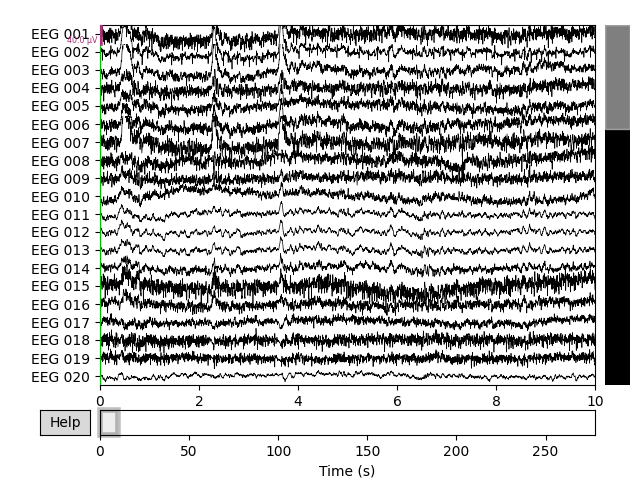•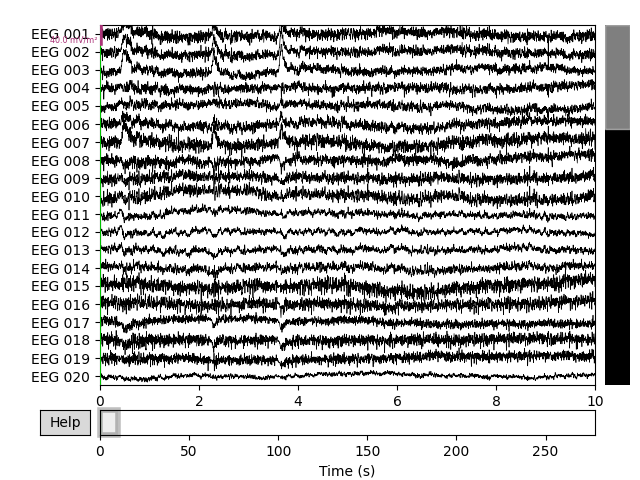Out:

Fitted sphere radius:         91.0 mm
Origin head coordinates:      -4.1 16.0 51.7 mm
Origin device coordinates:    1.4 17.8 -10.3 mm


Also look at the power spectral densities:

raw.plot_psd()
raw_csd.plot_psd()

•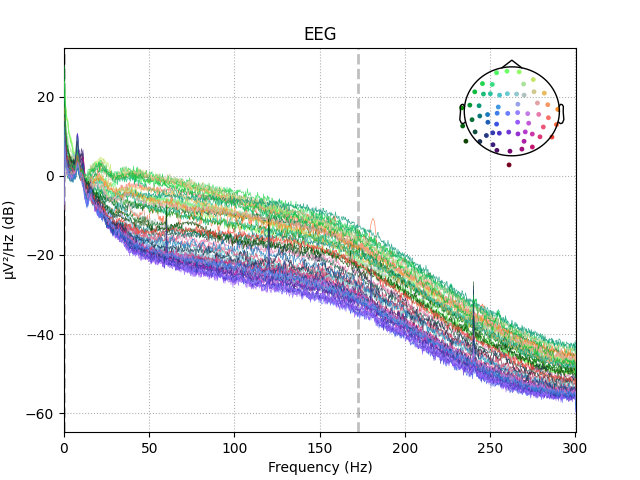•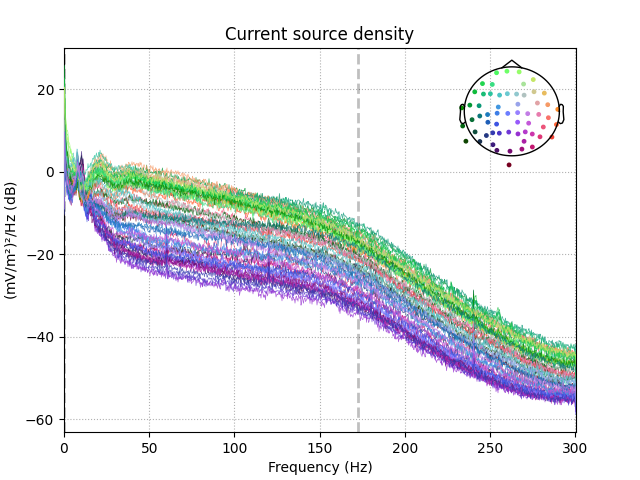Out:

Effective window size : 3.410 (s)
Effective window size : 3.410 (s)


CSD can also be computed on Evoked (averaged) data. Here we epoch and average the data so we can demonstrate that.

event_id = {'auditory/left': 1, 'auditory/right': 2, 'visual/left': 3,
'visual/right': 4, 'smiley': 5, 'button': 32}
epochs = mne.Epochs(raw, events, event_id=event_id, tmin=-0.2, tmax=.5,
evoked = epochs['auditory'].average()


Out:

Not setting metadata
320 matching events found
Applying baseline correction (mode: mean)
Created an SSP operator (subspace dimension = 1)
1 projection items activated


First let’s look at how CSD affects scalp topography:

times = np.array([-0.1, 0., 0.05, 0.1, 0.15])
evoked_csd = mne.preprocessing.compute_current_source_density(evoked)
evoked.plot_joint(title='Average Reference', show=False)
evoked_csd.plot_joint(title='Current Source Density')

•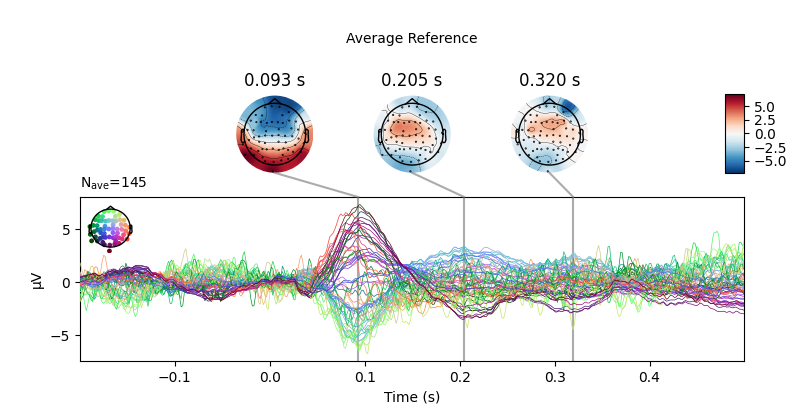•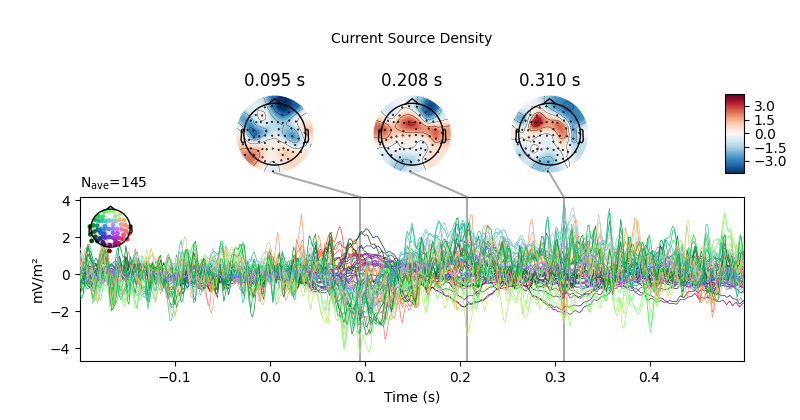Out:

Fitted sphere radius:         91.0 mm
Origin head coordinates:      -4.1 16.0 51.7 mm
Origin device coordinates:    1.4 17.8 -10.3 mm
Projections have already been applied. Setting proj attribute to True.
Projections have already been applied. Setting proj attribute to True.


CSD has parameters stiffness and lambda2 affecting smoothing and spline flexibility, respectively. Let’s see how they affect the solution:

fig, ax = plt.subplots(4, 4)
fig.set_size_inches(10, 10)
for i, lambda2 in enumerate([0, 1e-7, 1e-5, 1e-3]):
for j, m in enumerate([5, 4, 3, 2]):
this_evoked_csd = mne.preprocessing.compute_current_source_density(
evoked, stiffness=m, lambda2=lambda2)
this_evoked_csd.plot_topomap(
0.1, axes=ax[i, j], outlines='skirt', contours=4, time_unit='s',
colorbar=False, show=False)
ax[i, j].set_title('stiffness=%i\nλ²=%s' % (m, lambda2))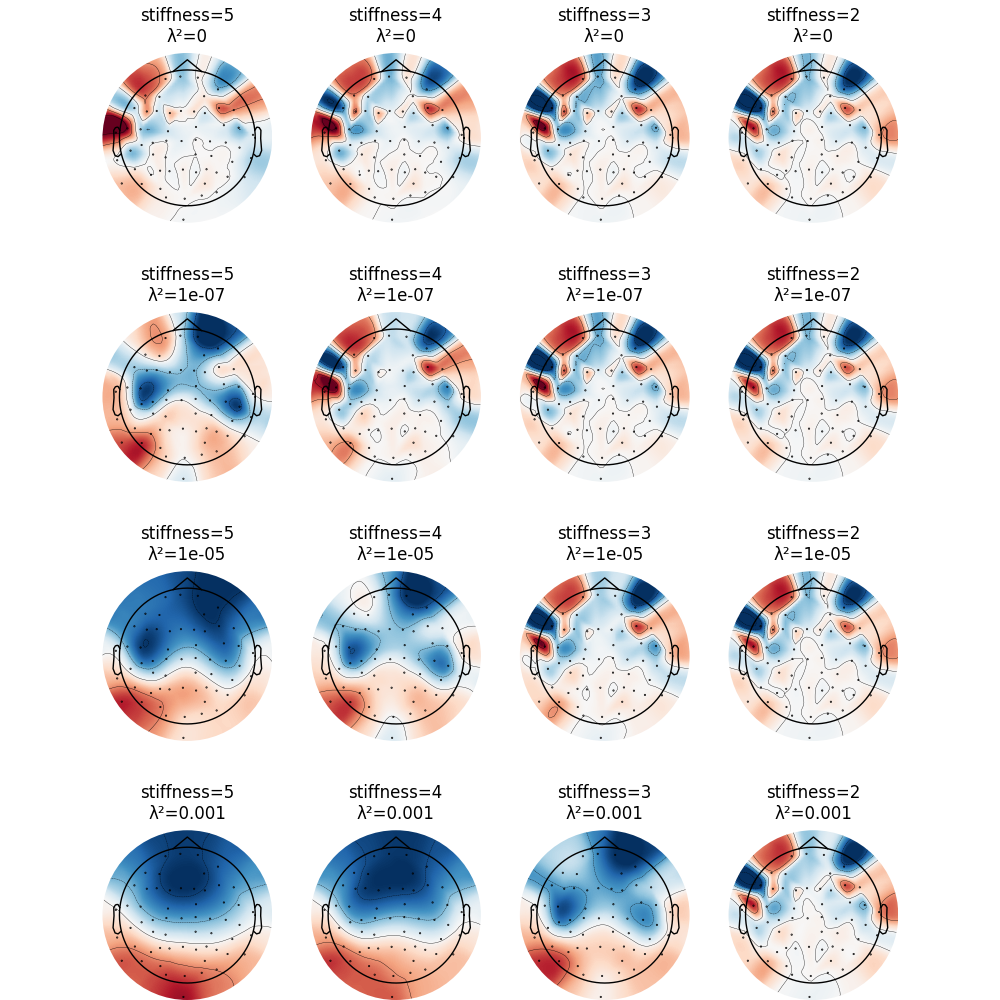Out:

Fitted sphere radius:         91.0 mm
Origin head coordinates:      -4.1 16.0 51.7 mm
Origin device coordinates:    1.4 17.8 -10.3 mm
Origin head coordinates:      -4.1 16.0 51.7 mm
Origin device coordinates:    1.4 17.8 -10.3 mm
Origin head coordinates:      -4.1 16.0 51.7 mm
Origin device coordinates:    1.4 17.8 -10.3 mm
Origin head coordinates:      -4.1 16.0 51.7 mm
Origin device coordinates:    1.4 17.8 -10.3 mm
Origin head coordinates:      -4.1 16.0 51.7 mm
Origin device coordinates:    1.4 17.8 -10.3 mm
Origin head coordinates:      -4.1 16.0 51.7 mm
Origin device coordinates:    1.4 17.8 -10.3 mm
Origin head coordinates:      -4.1 16.0 51.7 mm
Origin device coordinates:    1.4 17.8 -10.3 mm
Origin head coordinates:      -4.1 16.0 51.7 mm
Origin device coordinates:    1.4 17.8 -10.3 mm
Origin head coordinates:      -4.1 16.0 51.7 mm
Origin device coordinates:    1.4 17.8 -10.3 mm
Origin head coordinates:      -4.1 16.0 51.7 mm
Origin device coordinates:    1.4 17.8 -10.3 mm
Origin head coordinates:      -4.1 16.0 51.7 mm
Origin device coordinates:    1.4 17.8 -10.3 mm
Origin head coordinates:      -4.1 16.0 51.7 mm
Origin device coordinates:    1.4 17.8 -10.3 mm